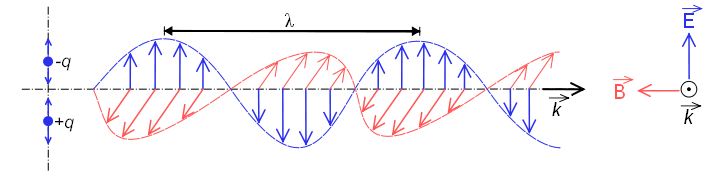Spectroscopy studies the interaction between electromagnetic radiation and matter. In this interaction, electromagnetic radiation can behave as a wave or as a particle, although no physical phenomenon has been observed in which both behaviors occur simultaneously.

When it behaves as a wave, it is made up of an electric field and a magnetic field that oscillate perpendicular and propagate at the speed of light $c=300000\;km/s$The electromagnetic spectrum classifies the different electromagnetic radiations according to their wavelength or frequency. Radio waves are those with the longest wavelength and lowest frequency, at the other extreme we find gamma rays, being the radiation with the shortest wavelength and highest frequency.The wavelength $\lambda$ is defined as the distance between two successive maximums or minimums. This distance is called a cycle.\\ The frequency $\nu"$ is defined as the number of cycles that pass through a point in space in one second. The unit of frequency is cycles/s hertz.

Wavelength and frequency are related to each other by the expression: \begin{equation} \lambda =\frac{c}{\nu} \end{equation} where $c$ is the speed of light.

In its corpuscular behavior, electromagnetic radiation interacts with matter in the form of particles called photons, whose energy is given by Planck's equation. \begin{equation} E=h\nu \end{equation} where $h=6.6262\times 10^{-34}\;Js$ is Planck's constant.